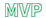# QlikView Scripting

Discussion Board for collaboration on QlikView Scripting.

Highlighted
New Contributor III

## File Code Issues

Hello All,

I am experiencing an issue trying to read a file from a third party into QV. The original file was able to be read in with (txt, codepage is 1252, embedded labels, delimiter is ',', msq); but at some point the file format was changed and can only be read with (txt, unicode, embedded labels, delimiter is ',', msq); . I am now trying to test some historical data and  cannot read both type of files at the same time. I have always read it using a loop so to load both files using different Character Sets. Is there a way to solution this issue? Any help with this matter is much appreciated.

Labels (3)

• ### Scripting

3 RepliesPartner

## Re: File Code Issues

Try the suggestion Rob posted in this thread.MVP

I deal with the same problem in my Script Log Analyzer tool. You can download the tool and see how I handle it in the script (see tab "Initial Logdata Load"):

What I do is test the first character of the logfile with various encodings to see if I can interpret it as a number. When I get a positive I use that encoding to read the file. Probably would break if someone used a MMM-DD-YYYY format, but haven't had a complaint (yet). Here's the relevant code that determines the encoding.

```FOR EACH vEncoding IN 'utf8', 'unicode', 'codepage is 1252';

TempCode:

FIRST 1 // Log first row

LOAD IsNum(left(@1:n,1)) as X // Test first char. Set to true if it's a number.

FROM

[\$(_logpath)]

(fix, \$(vEncoding))

;

EXIT FOR WHEN peek('X'); // If a number, assume we have the right encoding.

NEXT vEncoding;```Partner

## Re: File Code Issues

see Rob Wunderlich's post in this thread

"What I do is test the first character of the logfile with various encodings  to see if I can interpret it as a number. When I get a positive I use that encoding to read the file. Probably would break if someone used a MMM-DD-YYYY format, but haven't had a complaint (yet). Here's the relevant code that determines the encoding."

```FOR EACH vEncoding IN 'utf8', 'unicode', 'codepage is 1252';

TempCode:

FIRST 1 // Log first row

LOAD IsNum(left(@1:n,1)) as X // Test first char. Set to true if it's a number.

FROM

[\$(_logpath)]

(fix, \$(vEncoding))

;

EXIT FOR WHEN peek('X'); // If a number, assume we have the right encoding.

NEXT vEncoding;```MVP & Luminary

## Re: File Code Issues

I handle multiple files with different encodings in the Script Log Analyzer tool. You can take a look at the script.

The script bit that determines the encoding looks like this:

FOR EACH vEncoding IN 'utf8', 'unicode', 'codepage is 1252';
TempCode:
FIRST 1 // Log first row
LOAD IsNum(left(@1:n,1)) as X // Test first char. Set to true if it's a number.
FROM
[\$(_logpath)]
(fix, \$(vEncoding))
WHERE RecNo()>1 // Skip rec 1, which causes problems if it has a BOM (Byte Order Marker)
;
EXIT FOR WHEN peek('X'); // If a number, assume we have the right encoding.
NEXT vEncoding;

DROP TABLE TempCode;

and then \$(vEncoding) is used in the LOAD statement.  You may have to adjust the "IsNum(" test for your data. I know I'm looking for a number in that position, you may have to look at something specific to your data.  If it's something you can recognize, then you have the right codepage for that file.